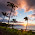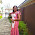# Basic Linux Shell Scripting Language - Arithmetic Operations

This is the third article on Basic Linux Shell Scripting Language. Before this article, I had published two articles on the said topic, which are:
I would recommend that, before you go through this article directly, please read this article so that it would become easier for you to understand.

In this article on Basic Linux Shell Scripting Language, we will be dealing with Mathematics. I will try to cover how basic operations in Mathematics are implemented in Shell Scripting along with some scripts which incorporate their uses. Most of these scripts are self explanatory.

Here we go !

### 1. Addition of Two Numbers

Here, we will need two variables, say x and y, to store two numbers and a third variable 'sum' to store their addition. Create a file 'Add.sh' , make it executable with chmod command and run it.

``````#!/bin/bash
echo "Enter First Number: "
read x
echo "Enter Second Number: "
read y
sum=\$(expr "\$x" + "\$y")
echo \$x + \$y = \$sum
``````
Output:

``````MyLinuxBox root ~ > chmod 744 add.sh
MyLinuxBox root ~ > ./add.sh
Enter First Number:
36
Enter Second Number:
24
36 + 24 = 60
MyLinuxBox root ~ >
``````

### 2. Subtraction of Two Numbers

This script 'Sub.sh' will be very much similar to the Addition script. In this script also, we will need two variables to store two inputs and the third variable 'diff' to hold the result 'x-y'.

``````#!/bin/bash
echo "Enter First Number: "
read x
echo "Enter Second Number: "
read y
diff=\$(expr "\$x" - "\$y")
echo \$x - \$y = \$diff
``````
Output:

``````MyLinuxBox root ~ > ./diff.sh
Enter First Number:
36
Enter Second Number:
24
36 - 24 = 12
MyLinuxBox root ~ >
``````

### 3. Multiplication of Two Numbers

We call this script as 'Mul.sh' and we will use the variable 'product' to store the result 'x * y'. Just remember to escape the '*' here.

``````#!/bin/bash
echo "Enter First Number: "
read x
echo "Enter Second Number: "
read y
product=\$(expr "\$x" \* "\$y")
echo "\$x * \$y = \$product "
``````
Output:

``````MyLinuxBox root ~ > ./mul.sh
Enter First Number:
50
Enter Second Number:
12
50 * 12 = 600
MyLinuxBox root ~ >
``````

### 4. Division of Two Numbers

Lets now create a file 'Div.sh', we will use the variable 'div' to store the result 'x / y'. Again, we need to escape '/' in the script.

``````#!/bin/bash
echo "Enter First Number: "
read x
echo "Enter Second Number: "
read y
div=\$(expr "\$x" / "\$y")
echo "\$x / \$y = \$div "
``````
Output:

``````MyLinuxBox root ~ > ./div.sh
Enter First Number:
243
Enter Second Number:
9
243 / 9 = 27
MyLinuxBox root ~ >
``````

### 5. Multiplication Table of a Number

With the knowledge of basic mathematical operations, time for a Level Up! This time, we will write a script that will print multiplication table of any number. For this, we need a For loop and `seq` command. `seq` command will just generate sequence of numbers (1 to 10 in this case).

``````#!/bin/bash
echo "Enter the number: "
read num

for i in \$(seq 1 10)
do
j=\$(expr \$i \* \$num)
echo \$j
done
``````
Output:

``````Enter the number:
17
17
34
51
68
85
102
119
136
153
170
``````

### 6. Check Whether the Number is Even or Odd

This time, we will make use of a 'Modulo' operator and one 'If-then-else' statement.

``````#!/bin/bash
echo "Enter the number: "
read num

i=\$(expr \$num % 2)

if [ \$i -eq 0 ]
then
echo "\$num is Even"
else
echo "\$num is Odd"
fi
``````
Output:

``````MyLinuxBox root ~ > ./EvenOdd.sh
Enter the number:
45
45 is Odd
MyLinuxBox root ~ > ./EvenOdd.sh
Enter the number:
78
78 is Even
MyLinuxBox root ~ >
``````

### 7. Find the Factorial of a Number

Now, let us create a script which calculates factorial of a given number using a 'while' loop.

``````#!/bin/bash
echo "Enter the number: "
read num
fact=1

while [ \$num -gt 0 ]
do
fact=\$(expr \$fact \* \$num)
num=\$(expr \$num - 1)
done

echo \$fact
``````
Output:

``````MyLinuxBox root ~ > ./Fact.sh
Enter the number:
7
5040
MyLinuxBox root ~ >
``````

### 8. Determine Whether a Number is Prime or Not

This script will need both the loop statement and conditional statement.

``````#!/bin/bash
echo "Enter the number: "
read num
c=1

if [ \$num -eq 1 ]
then
echo "Its Neither Prime nor Composite..!"
else
i=2
while [ \$i -le \$num ]
do
j=\$(expr \$num % \$i)
if [ j -eq 0 ]
then
c=\$(expr \$c + 1)
fi
i=\$(expr \$i + 1)
done

if [ c -eq 2 ]
then
echo "Yeah! Its Prime!"
else
echo "No! Its not Prime!"
fi
fi
``````
Output:

``````MyLinuxBox root ~ > ./Prime.sh
Enter the number:
1
Its Neither Prime nor Composite..!
MyLinuxBox root ~ > ./Prime.sh
Enter the number:
87
No! Its not Prime!
MyLinuxBox root ~ > ./Prime.sh
Enter the number:
23
Yeah! Its Prime!
MyLinuxBox root ~ >
``````
That's all! In this article, I tried to cover how basic mathematical operations are implemented in Shell Scripting along with some scripts which were based on those mathematical operations. For any feedback, please use the comment section below and stay tuned for some more articles on Basic Linux Shell Scripting Language.

#### 3 comments:

1.Hi, it seems to me that the function "expr" does not work if "x" and "y" are decimal numbers... does it only work for integers?

2.That's right. Using "expr", you can perform operations only on integers. In case you have to perform operations on decimals, you cal use "bc".

3.How do I add up even number, e.g even now. Between1to 10